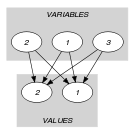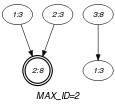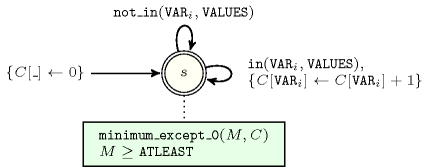## 5.58. cardinality_atleast

Origin
Constraint

$\mathrm{𝚌𝚊𝚛𝚍𝚒𝚗𝚊𝚕𝚒𝚝𝚢}_\mathrm{𝚊𝚝𝚕𝚎𝚊𝚜𝚝}\left(\mathrm{𝙰𝚃𝙻𝙴𝙰𝚂𝚃},\mathrm{𝚅𝙰𝚁𝙸𝙰𝙱𝙻𝙴𝚂},\mathrm{𝚅𝙰𝙻𝚄𝙴𝚂}\right)$

Arguments
 $\mathrm{𝙰𝚃𝙻𝙴𝙰𝚂𝚃}$ $\mathrm{𝚍𝚟𝚊𝚛}$ $\mathrm{𝚅𝙰𝚁𝙸𝙰𝙱𝙻𝙴𝚂}$ $\mathrm{𝚌𝚘𝚕𝚕𝚎𝚌𝚝𝚒𝚘𝚗}\left(\mathrm{𝚟𝚊𝚛}-\mathrm{𝚍𝚟𝚊𝚛}\right)$ $\mathrm{𝚅𝙰𝙻𝚄𝙴𝚂}$ $\mathrm{𝚌𝚘𝚕𝚕𝚎𝚌𝚝𝚒𝚘𝚗}\left(\mathrm{𝚟𝚊𝚕}-\mathrm{𝚒𝚗𝚝}\right)$
Restrictions
 $\mathrm{𝙰𝚃𝙻𝙴𝙰𝚂𝚃}\ge 0$ $\mathrm{𝙰𝚃𝙻𝙴𝙰𝚂𝚃}\le |\mathrm{𝚅𝙰𝚁𝙸𝙰𝙱𝙻𝙴𝚂}|$ $\mathrm{𝚛𝚎𝚚𝚞𝚒𝚛𝚎𝚍}$$\left(\mathrm{𝚅𝙰𝚁𝙸𝙰𝙱𝙻𝙴𝚂},\mathrm{𝚟𝚊𝚛}\right)$ $\mathrm{𝚛𝚎𝚚𝚞𝚒𝚛𝚎𝚍}$$\left(\mathrm{𝚅𝙰𝙻𝚄𝙴𝚂},\mathrm{𝚟𝚊𝚕}\right)$ $\mathrm{𝚍𝚒𝚜𝚝𝚒𝚗𝚌𝚝}$$\left(\mathrm{𝚅𝙰𝙻𝚄𝙴𝚂},\mathrm{𝚟𝚊𝚕}\right)$
Purpose

$\mathrm{𝙰𝚃𝙻𝙴𝙰𝚂𝚃}$ is the minimum number of time that a value of $\mathrm{𝚅𝙰𝙻𝚄𝙴𝚂}$ is taken by the variables of the collection $\mathrm{𝚅𝙰𝚁𝙸𝙰𝙱𝙻𝙴𝚂}$.

Example
$\left(1,〈3,3,8〉,〈3,8〉\right)$

In this example, values 3 and 8 are respectively used 2, and 1 times. The $\mathrm{𝚌𝚊𝚛𝚍𝚒𝚗𝚊𝚕𝚒𝚝𝚢}_\mathrm{𝚊𝚝𝚕𝚎𝚊𝚜𝚝}$ constraint holds since its first argument $\mathrm{𝙰𝚃𝙻𝙴𝙰𝚂𝚃}=1$ is assigned to the minimum number of time that values 3 and 8 occur in the collection $〈3,3,8〉$.

Typical
 $\mathrm{𝙰𝚃𝙻𝙴𝙰𝚂𝚃}>0$ $\mathrm{𝙰𝚃𝙻𝙴𝙰𝚂𝚃}<|\mathrm{𝚅𝙰𝚁𝙸𝙰𝙱𝙻𝙴𝚂}|$ $|\mathrm{𝚅𝙰𝚁𝙸𝙰𝙱𝙻𝙴𝚂}|>1$ $|\mathrm{𝚅𝙰𝙻𝚄𝙴𝚂}|>0$ $|\mathrm{𝚅𝙰𝚁𝙸𝙰𝙱𝙻𝙴𝚂}|>|\mathrm{𝚅𝙰𝙻𝚄𝙴𝚂}|$
Symmetries
• Items of $\mathrm{𝚅𝙰𝚁𝙸𝙰𝙱𝙻𝙴𝚂}$ are permutable.

• Items of $\mathrm{𝚅𝙰𝙻𝚄𝙴𝚂}$ are permutable.

• An occurrence of a value of $\mathrm{𝚅𝙰𝚁𝙸𝙰𝙱𝙻𝙴𝚂}.\mathrm{𝚟𝚊𝚛}$ that does not belong to $\mathrm{𝚅𝙰𝙻𝚄𝙴𝚂}.\mathrm{𝚟𝚊𝚕}$ can be replaced by any other value that also does not belong to $\mathrm{𝚅𝙰𝙻𝚄𝙴𝚂}.\mathrm{𝚟𝚊𝚕}$.

• All occurrences of two distinct values in $\mathrm{𝚅𝙰𝚁𝙸𝙰𝙱𝙻𝙴𝚂}.\mathrm{𝚟𝚊𝚛}$ or $\mathrm{𝚅𝙰𝙻𝚄𝙴𝚂}.\mathrm{𝚟𝚊𝚕}$ can be swapped; all occurrences of a value in $\mathrm{𝚅𝙰𝚁𝙸𝙰𝙱𝙻𝙴𝚂}.\mathrm{𝚟𝚊𝚛}$ or $\mathrm{𝚅𝙰𝙻𝚄𝙴𝚂}.\mathrm{𝚟𝚊𝚕}$ can be renamed to any unused value.

Arg. properties

Functional dependency: $\mathrm{𝙰𝚃𝙻𝙴𝙰𝚂𝚃}$ determined by $\mathrm{𝚅𝙰𝚁𝙸𝙰𝙱𝙻𝙴𝚂}$ and $\mathrm{𝚅𝙰𝙻𝚄𝙴𝚂}$.

Usage

An application of the $\mathrm{𝚌𝚊𝚛𝚍𝚒𝚗𝚊𝚕𝚒𝚝𝚢}_\mathrm{𝚊𝚝𝚕𝚎𝚊𝚜𝚝}$ constraint is to enforce a minimum use of values.

Remark

This is a restricted form of a variant of an $\mathrm{𝚊𝚖𝚘𝚗𝚐}$ constraint and of the $\mathrm{𝚐𝚕𝚘𝚋𝚊𝚕}_\mathrm{𝚌𝚊𝚛𝚍𝚒𝚗𝚊𝚕𝚒𝚝𝚢}$ constraint. In the original $\mathrm{𝚐𝚕𝚘𝚋𝚊𝚕}_\mathrm{𝚌𝚊𝚛𝚍𝚒𝚗𝚊𝚕𝚒𝚝𝚢}$ constraint, one specifies for each value its minimum and maximum number of occurrences.

Algorithm

generalisation: $\mathrm{𝚐𝚕𝚘𝚋𝚊𝚕}_\mathrm{𝚌𝚊𝚛𝚍𝚒𝚗𝚊𝚕𝚒𝚝𝚢}$ (single $\mathrm{𝚌𝚘𝚞𝚗𝚝}\mathrm{𝚟𝚊𝚛𝚒𝚊𝚋𝚕𝚎}$ replaced by an individual $\mathrm{𝚌𝚘𝚞𝚗𝚝}\mathrm{𝚟𝚊𝚛𝚒𝚊𝚋𝚕𝚎}$ for each value).

Keywords
Arc input(s)

$\mathrm{𝚅𝙰𝚁𝙸𝙰𝙱𝙻𝙴𝚂}$ $\mathrm{𝚅𝙰𝙻𝚄𝙴𝚂}$

Arc generator
$\mathrm{𝑃𝑅𝑂𝐷𝑈𝐶𝑇}$$↦\mathrm{𝚌𝚘𝚕𝚕𝚎𝚌𝚝𝚒𝚘𝚗}\left(\mathrm{𝚟𝚊𝚛𝚒𝚊𝚋𝚕𝚎𝚜},\mathrm{𝚟𝚊𝚕𝚞𝚎𝚜}\right)$

Arc arity
Arc constraint(s)
$\mathrm{𝚟𝚊𝚛𝚒𝚊𝚋𝚕𝚎𝚜}.\mathrm{𝚟𝚊𝚛}\ne \mathrm{𝚟𝚊𝚕𝚞𝚎𝚜}.\mathrm{𝚟𝚊𝚕}$
Graph property(ies)
$\mathrm{𝐌𝐀𝐗}_\mathrm{𝐈𝐃}$$=|\mathrm{𝚅𝙰𝚁𝙸𝙰𝙱𝙻𝙴𝚂}|-\mathrm{𝙰𝚃𝙻𝙴𝙰𝚂𝚃}$

Graph class
 $•$$\mathrm{𝙰𝙲𝚈𝙲𝙻𝙸𝙲}$ $•$$\mathrm{𝙱𝙸𝙿𝙰𝚁𝚃𝙸𝚃𝙴}$ $•$$\mathrm{𝙽𝙾}_\mathrm{𝙻𝙾𝙾𝙿}$

Graph model

Using directly the graph property $\mathrm{𝐌𝐈𝐍}_\mathrm{𝐈𝐃}$ $=$ $\mathrm{𝙰𝚃𝙻𝙴𝙰𝚂𝚃}$, and replacing the disequality of the arc constraint by an equality does not work since it ignores values that are not assigned to any variable. This comes from the fact that isolated vertices are removed from the final graph.

Parts (A) and (B) of Figure 5.58.1 respectively show the initial and final graph associated with the Example slot. Since we use the $\mathrm{𝐌𝐀𝐗}_\mathrm{𝐈𝐃}$ graph property, the vertex with the maximum number of predecessor (i.e., namely two predecessors) is stressed with a double circle. As a consequence the first argument $\mathrm{𝙰𝚃𝙻𝙴𝙰𝚂𝚃}$ of the $\mathrm{𝚌𝚊𝚛𝚍𝚒𝚗𝚊𝚕𝚒𝚝𝚢}_\mathrm{𝚊𝚝𝚕𝚎𝚊𝚜𝚝}$ constraint is assigned to the total number of variables 3 minus 2.

##### Figure 5.58.1. Initial and final graph of the $\mathrm{𝚌𝚊𝚛𝚍𝚒𝚗𝚊𝚕𝚒𝚝𝚢}_\mathrm{𝚊𝚝𝚕𝚎𝚊𝚜𝚝}$ constraint(a) (b)
Automaton

Figure 5.58.2 depicts the automaton associated with the $\mathrm{𝚌𝚊𝚛𝚍𝚒𝚗𝚊𝚕𝚒𝚝𝚢}_\mathrm{𝚊𝚝𝚕𝚎𝚊𝚜𝚝}$ constraint. To each variable ${\mathrm{𝚅𝙰𝚁}}_{i}$ of the collection $\mathrm{𝚅𝙰𝚁𝙸𝙰𝙱𝙻𝙴𝚂}$ corresponds a 0-1 signature variable ${S}_{i}$. The following signature constraint links ${\mathrm{𝚅𝙰𝚁}}_{i}$ and ${S}_{i}$: ${\mathrm{𝚅𝙰𝚁}}_{i}\in \mathrm{𝚅𝙰𝙻𝚄𝙴𝚂}⇔{S}_{i}$.

##### Figure 5.58.2. Automaton of the $\mathrm{𝚌𝚊𝚛𝚍𝚒𝚗𝚊𝚕𝚒𝚝𝚢}_\mathrm{𝚊𝚝𝚕𝚎𝚊𝚜𝚝}$ constraint